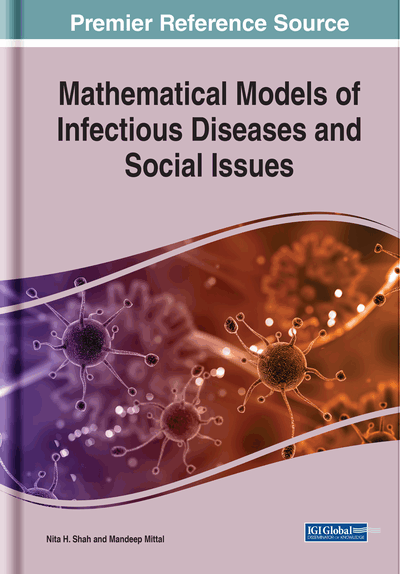# Analysis of Discrete System Modelling Followed by Spread of Infectious Diseases Problem in Fuzzy Environments

Abdul Alamin (Maulana Abul Kalam Azad University of Technology, West Bengal, India), Sankar Prasad Mondal (Maulana Abul Kalam Azad University of Technology, West Bengal, India), Kunal Biswas (Maulana Abul Kalam Azad University of Technology, West Bengal, India) and Shariful Alam (Indian Institute of Engineering Science and Technology, Shibpur, India)
DOI: 10.4018/978-1-7998-3741-1.ch006

## Abstract

In this chapter, the authors discuss the solution of spread of infectious diseases in terms of SI model in fuzzy environment, which is modelled in a typical discrete system. As the system is discrete in nature, the concept of difference equation has been embarked. In order to understand the underlying uncertainty perspective, they explored the fuzzy difference equations to study the problem.
Chapter Preview
Top

## Introduction

### Fuzzy Set Theory

Due to inherent uncertainty of the preference expressions, real-world decision-making activities, management, storage and the extraction of various useful information is not always presented as crisp numbers. It is understood that fuzzy numbers are a significant player in evaluating information systems (Altman, D. 1994). Machine learning, un-certainty mining and associated information systems are established by Fuzzy set theory (Altman, D. 1994). Membership degree in the fuzzy set theory employes a single crisp value within 1\2 0;1. It is understood that classy fuzzy set experiences limitations while working with incomplete and uncertain information’s. Due to such limitations, additional generalisations of fuzzy sets were developed (Kosiński, W. 2006).

### Fuzzy Epidemic Modelling

The epidemic modelling of the disease model comprises several parameters which are uncertain and heterogeneous in the population mass (Mu, X., Zhang, Q., & Rong, L. 2019). Different dynamical systems in the epidemic models include the application to fuzzy theory approach (Verma, R., Tiwari, S. P., & Upadhyay, R. K. 2019). Several fuzzy parameters in the disease model include the interval value systems which is in a prey-predator model which thereby finds application in the computer network Mishra, B. K., & Pandey, S. K. (2010). Also, the dynamical behaviour of an epidemic modelling with fuzzy transmission has been explained by (Jafelice, R. M., Pereira, B. L., Bertone, A. M. A., & Barros, L. C. 2019). Human disease transmission in the fuzzy epidemic modelling has been studied by (Bufford, J. L., Hulme, P. E., Sikes, B. A., Cooper, J. A., Johnston, P. R., & Duncan, R. P. 2019).

## Complete Chapter List

Search this Book:
Reset